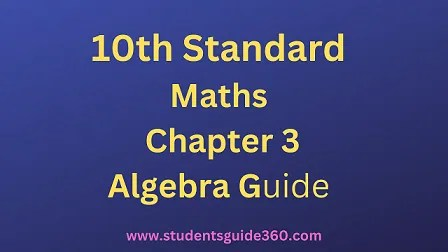# 10th Maths Guide Chapter 3. Algebra Exercise 3.5

10th Standard Maths Chapter 3 Algebra Samacheer Kalvi Guide Exercise 3.5 Book Back Answers Solutions. TN 10th SSLC Samacheer Kalvi Guide. 10th All Subject Guide – Click Here. Class 1 to 12 All Subject Book Back Answers – Click Here## 10th Maths Guide Chapter 3. Algebra Exercise 3.5

Solution:

Solution:

Solution:

Solution:

### 5.If a polynomial p(x) = x2 – 5x – 14 is divided by another polynomial q(x) we get x−7 / x+2 find q(x).

Solution:
p(x) = x2 – 5x – 14

……..